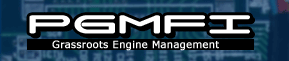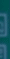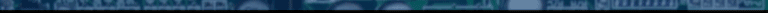Library> UnderstandingMaps Destinations: Home | Library | Change Log | IndexSearch | Go

## Understanding Fuel and Ignition Maps.

Fuel Maps

Below around 80% throttle, the ECU uses the oxygen sensor in conjunction with the fuel tables to calculate how much fuel is needed for your engine. This is called "closed loop" mode because the ECU is in a closed feedback loop using the Oxygen Sensor to steer the Air Fuel Ratio to the desired level. Above the throttle position threshold, the ECU exclusively uses look up tables. The ECU looks through the lookup tables and, depending on engine speed and intake manifold vacuum, selects a length of time that the injectors will stay open. If you are interested in modifying your ECU, chances are that your ECU spends a fair amount of time in the look up table mode (open loop).

I will assume you are looking at an editor like this:The columns are split up into small segments based on intake manifold vacuum. The left most column is the most vacuum the ECU accounts for (shut throttle plate) and the right is obviously WOT. The OBD0 ECUs have 15 of these columns and use inches of mercury as a unit, typically. The OBD1 ECU's use 10 columns and use millibar (mBar) as a unit. The editor can choose to display this information in two ways. Absolute Pressure or Relative Pressure.

The rows are split up into little rev ranges.

The values in the cells are in what is believed to be milliseconds that the injector stays open. To calculate these values the editors use a formula (in this case: OBD0 Fuel; OBD1_8bit Fuel for OBD1 applications) that combines the raw 8 bit value at the location of the cell in the map with a Column Multiplier to arrive at the value you see in the editor. Some editors allow you to directly manipulate the raw value directly instead of interpreting it as a pulse-width

Ignition Maps

The ECU also does the look up table thing with the ignition maps.Again, the columns are vacuum and the rows are RPM. The value in the cells is degrees of ignition advance.

Interpolation

When choosing a value from any of the fuel or ignition tables, the ECU interpolates between the currently used cell and surrounding cells to ensure smoothness. For example, lets say you are at 5775 RPM and 20 inhg (in the above maps). Your engine is in between column 3 and 4 and row 7 and 8 in the above tables. To find the actual value the ecu is using, you can go through essentially the same process that the ECU uses. The ECU code is actually doing a weighted average between the values in the cells (3,7) and (4,7) based on the map sensors value, then the same for (3,8) and (4,8) again with the map sensor, and then those values are averaged together based on RPM. Now for some math:

Our process will be as follows:
Interpolate column wise for the current cell and the east cell.
Interpolate column wise for the south cell and the southeast cell.
Take values calculated above and interpolate between them in a row wise fashion.

The data the fuel map provides:
(3,7) = 21 inhg, 5500 rpm, val = 2.16
(4,7) = 19 inhg, 5500 rpm, val = 2.56
(3,8) = 21 inhg, 6050 rpm, val = 2.25
(4,8) = 19 inhg, 6050 rpm, val = 2.62

//notice that we are half way inbetween the rows
//and halfway inbetween the columns
rpmval = 5775
mapval = 20 inhg

First we calc the column interpolation for (3,7) and (4,7):
(|cell'sColumnMapValue - mapval|)/intervalBetweenColumnValues * value = that cells x contribution

(21-20)/(21-19)*2.16 + ((20-19)/(21-19)*2.56) = .5 * 2.16 + .5 * 2.56 = 2.36

Then for (3,8) and (4,8):

(21-20)/(21-19)*2.25 + ((20-19)/(21-19)*2.62) = .5 * 2.25 + .5 * 2.62 = 2.435

Now, we do the row interpolation with our new values:
(|cell'sRowRPMValue - mapval|)/intervalBetweenRowValues * value = that cell's y contribution

(6050-5775)/(6050-5500)* 2.36 + (5775-5500)/(6050-5500) * 2.435 = .5* 2.36 + .5* 2.435 = 2.3975

The ECU would then use 2.3975

 Revision: r1.2 - 21 Nov 2005 - 19:26 GMT - ben ogle { Edit | Attach | History | More }Copyright © 2002-present by the contributing authors. All material on this collaboration platform is the property of the contributing authors, and is covered by the Non-Commercial Share-Alike License unless explicitly stated otherwise.
 Ideas, requests, problems regarding the PGMFI TWiki?? E-Mail the WikiAdmin Site Designed By: Digital Fusion   Need a website?